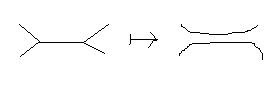# Is a knotted trivalent graph determined by its set of unzips?

A knotted trivalent graph (KTG) is a framed embedding of a trivalent graph in $\mathbb{R}^3$, modulo ambient isotopy. I believe they were first considered (in the quantum topological context, at least) in a paper by D. Thurston. There is an "unzip" move on edges, turning an "H" pattern into a pair of edges (Thanks to Kea for the hand-drawing of this image).A knotted theta graph unzips in three ways (one unzip for each edge), giving rise to three possible 2-component framed links, related to one another by handleslide (Kirby 2). The following statement looked obvious at first to me, but now I'm beginning to doubt it's even true, and I have no idea how to prove it or to find a counterexample. It `feels' well-known.

Question: Given two KTG's (say knotted theta graphs for simplicity), all of whose unzips coincide (i.e. given an edge e in one, there exists and edge f in the other, such that unzipping along e and along f give ambient isotopic results), does it follow that the KTG's are themselves ambient isotopic? Or do there exist distinct KTG's all of whose unzips coincide?
• If you wanted to investigate possible counterexamples, you could distinguish theta graphs up to ambient isotopy via the methods of Heard, Hodgson, Martelli, and Petronio (arxiv.org/abs/0804.4790), that is you could label each graph with appropriate integers (at a vertex with edges labeled by (a,b,c), 1/a+1/b+1/c>1) and associate a compact orbifold without boundardy to each embedding. Actually, you should associate 6 orbifolds to each embedding one for every permutation of (a,b,c). To create your own examples, you could use the software (ms.unimelb.edu.au/~snap/orb.html). – Neil Hoffman Feb 15 '13 at 10:48

A framed theta graph (ribbon surface) is really a thrice puncture sphere embedded in $S^3$ (pants). A stronger question is whether one may have two pants whose boundaries give the same 3-component link? In fact, suppose the interiors of the two pants are disjointly embedded in the link complement. The union is a genus 2 surface. Conversely, given a genus 2 surface, one may choose a pants decomposition of it to get such a pair. I claim that if one takes a genus 2 surface bounding an incompressible surface to one side (so the other side is compressible), a generic pants decomp will give inequivalent theta graphs. One may assume the link is hyperbolic without symmetries. If they were equivalent, there would be an isometry taking one pants to the other. This isometry would be finite order, giving a symmetry of the link complement, a contradiction. It remains to find such a surface and pants decomp, but I'm sure any sufficiently complicated asymmetric example will work.

• This is a very nice idea which should answer the question (after carrying out such a computation)- thank you! – Daniel Moskovich Feb 24 '13 at 23:31
• Thinking about this some more, I'm confused: We want the boundaries of the pants to give us the same 3-component <i>framed</i> link. How does this argument take framing into account? Isn't the framing different for the two sides? – Daniel Moskovich Feb 25 '13 at 1:30
• Since the pants are disjointly embedded, they induce the same framing on the boundary. – Ian Agol Feb 25 '13 at 4:18

This is really more of a comment, but here goes:

The question you ask is "dual" to this question: Suppose you have two theta graphs $\theta_1$ and $\theta_2$ such that for each edge $e_1$ of $\theta_1$, there is an edge $e_2$ of $\theta_2$ such that the knots $\theta_1 - e_1$ and $\theta_2 - e_2$ are isotopic. Must $\theta_1$ and $\theta_2$ be isotopic?

The answer to the dual question is "no" as seen by an unknotted theta graph and Kinoshita's graph.

In terms of suggestions for a places to look for counterexamples:

1. Maybe take a tunnel number 1, 2-component link with distinct unknotting tunnels. For each unknotting tunnel, form the graph which is the union of the link with the unknotting tunnel & then zip it to make a theta graph. Work of Cho & McCullough should tell you the answer to your question in this setting.

2. Take two (knotted) genus two handlebodies which are not isotopic in $\mathbb{R}^3$ but which have homeomorphic exteriors $X_1$ and $X_2$. The unzips correspond to attaching 2-handles to the boundaries of $X_1$ and $X_2$. If you could find the right sort of 2-handle additions to guarantee that the resulting links are isotopic (which seems like it might be hard, but not totally out of the question to me) you would have your counterexamples. The graphs produced would have the stronger property of not being even neighborhood isotopy equivalent. (I think it should follow from the various proofs of the "band sum theorem" (eg. by Scharlemann/Gabai) that this approach won't work if you try to create a split link with your unzip.)

A final remark: The prior comments ignore any sort of framing. Probably that just increases the likelihood of a counterexample.

• Thank you! I will try this- this sounds very promising in fact! – Daniel Moskovich Feb 20 '13 at 0:41

As a special case, consider a (framed) $\theta$ graph, such that one zipping contains an unknotted component and another zipping is a split link, then the $\theta$ graph is isotopic to a planar one. This follows from a theorem of Scharlemann, which states that if a band sum of knots is trivial, then the knots form a trivial link.